### Linear Vector Spaces: Change of Basis

#### Learning Outcomes

• Calculate the components of a vector in different ONBs

### Change of Basis

In this section, we will introduce the concept of transformation between coordinate systems. The components of vectors in a linear vector spaces depend on the chosen basis set of vectors. If an alternate basis set is chosen, the components of the vector change according to a transformation matrix that is calculated based on the relationship between the vectors in the initial basis set and those in the alternate basis set. Before the coordinate transformation matrices are introduced, we will introduce the Kronecker delta(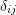), which is a tool that will shorten the derivations below. Bywe mean the following function ofand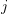: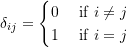Let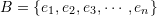be an orthonormal set of basis vectors in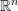. Then,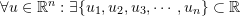such that: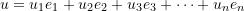The numbers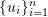are called the components of u in the orthonormal basis set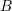and each component can be obtained using the dot product operation: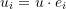Then, the vectorcan also be written as: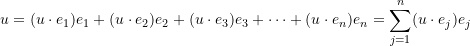Let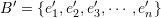be a different orthonormal set of basis vectors in, and let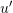be the representation of the vectorin the new coordinate system. In this case, we have a new set of components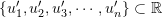such that: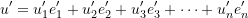In order to find the relationship between the componentsand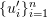we first find the relationship between the basis vectors. As a first step, each basis vector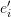will be expressed in terms of the original basis vectors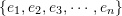as was done above for: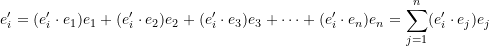Let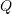denote the matrix of numbers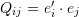. This matrix of numbers is the matrix of transformation between the components in the basis setsand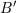. Let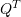denote the transpose of, i.e.: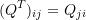We will now show that the rows ofandare orthonormal.
Indeed, since we know that the setis orthonormal, we have:i.e., if we multiply the components of the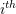row ofby the components of the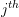row of, we get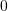when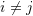and we get 1 when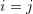.

If we use I to denote the identity matrix (i.e., with 1 in the diagonal components and 0 in the off diagonal components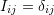) then we have: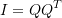By expressing the basis vectors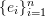in terms of the orthonormal basis set of vectorsand using the same argument above we have: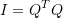Thus, the matrixis an example of a special type of operators (orthogonal matrices) which will be studied later. This matrix can be used to relate the components ofwith the components ofas follows: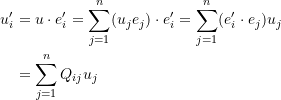This last relationship can be written in matrix form as follows. If u represents the components of a vector in the orthonormal basis setandrepresents the components of that vector in the orthonormal basis set, then, these components are related by the matrix: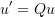The JSXgraph tool in the Matrix Representation and Change of Basis is a useful for the illustration of the concept and calculations of coordinate transformations in 2D.